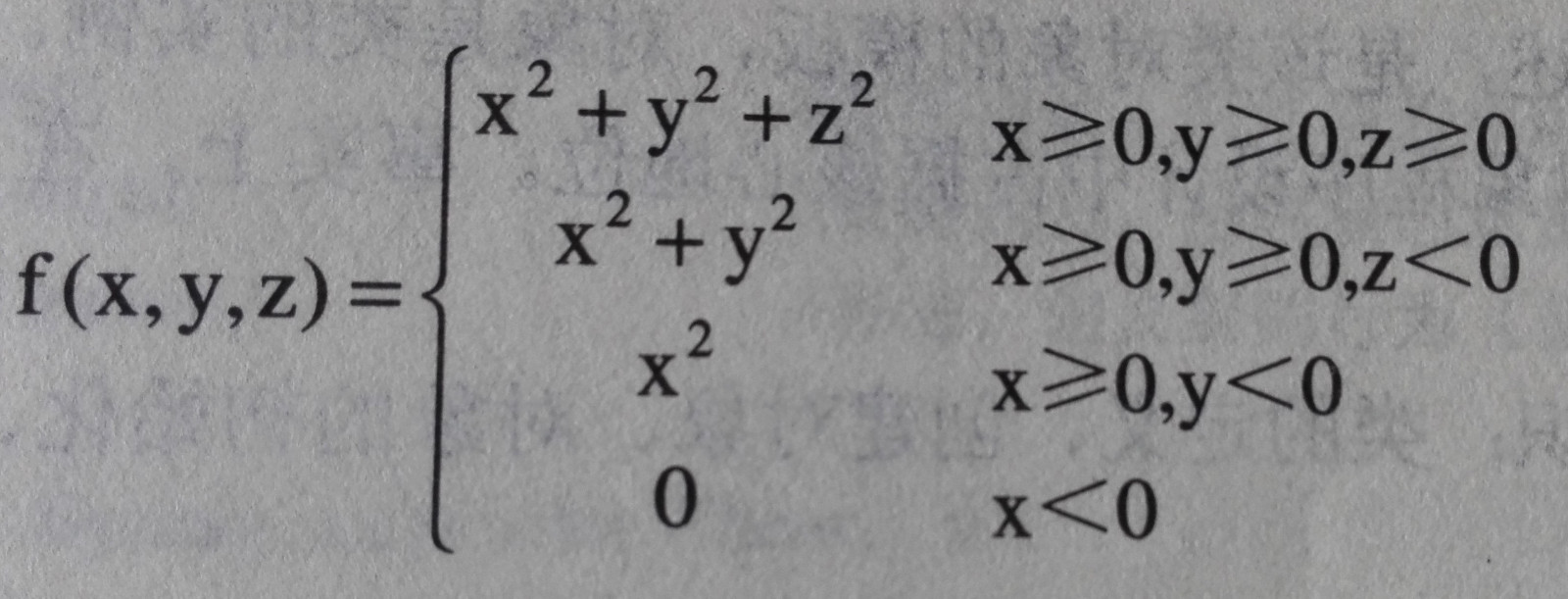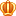##账号 自动登录 找回密码 密码 立即注册
 搜索

# [已解决]怎么写阿发表于 2023-11-21 08:31:52 | 显示全部楼层 |阅读模式

### 马上注册，结交更多好友，享用更多功能^_^

x【输入形式】-1 2 5
【输出形式】0
【样例输入】

【样例输出】
【样例说明】
【评分标准】2023-11-21 08:31:58
1. public class FormulaCalculator {
2.     public int f(int x, int y) {
3.         return x + y;
4.     }

5.     public int f(int x, int y, int z) {
6.         return x + y - z;
7.     }

8.     public static void main(String[] args) {
9.         FormulaCalculator calculator = new FormulaCalculator();
10.         int x = Integer.parseInt(args);
11.         int y = Integer.parseInt(args);
12.         int z = Integer.parseInt(args);
13.         int result = calculator.f(x, y, z);
14.         System.out.println(result);
15.     }
16. }发表于 2023-11-21 08:31:58 | 显示全部楼层    本楼为最佳答案
 .pct{min-height:100px;}public class FormulaCalculator {     public int f(int x, int y) {         return x + y;     }     public int f(int x, int y, int z) {         return x + y - z;     }     public static void main(String[] args) {         FormulaCalculator calculator = new FormulaCalculator();         int x = Integer.parseInt(args);         int y = Integer.parseInt(args);         int z = Integer.parseInt(args);         int result = calculator.f(x, y, z);         System.out.println(result);     } } 复制代码 这段代码定义了一个名为FormulaCalculator的类，其中包含了两个重载的f方法，分别接受两个和三个参数。在main方法中，通过命令行参数获取x，y，z的值，并调用f方法计算结果，最后输出结果。

 您需要登录后才可以回帖 登录 | 立即注册 本版积分规则 回帖后跳转到最后一页